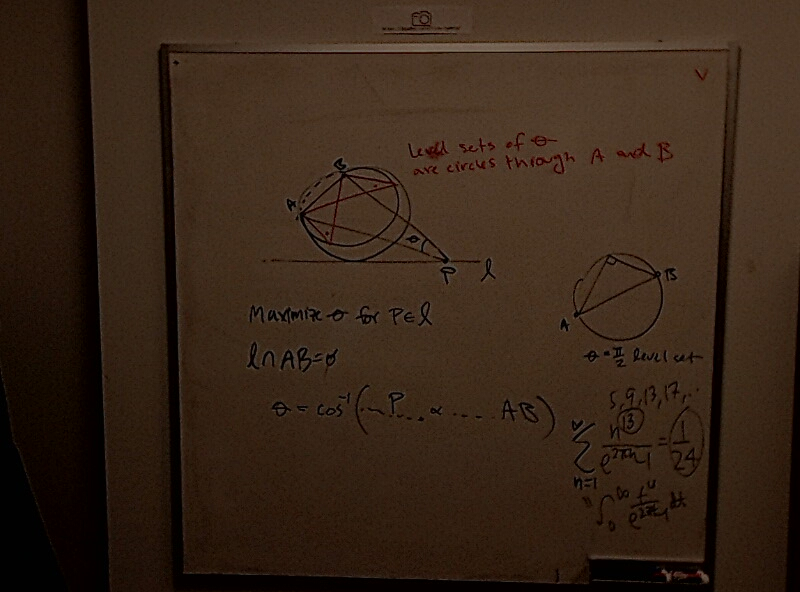# Optimization Doodles and Dave's Amazing Summation (Redo)

Nov 4, 2022Dave has an amazing summation: $\displaystyle \sum_{n=1}^\infty \frac{n^{13}}{e^{2\pi n} - 1} = \frac{1}{24}$

Moreover, this is exactly equal to the integral!

$\displaystyle \int_0^\infty \frac{x^{13}}{e^{2 \pi x} - 1} dx = \displaystyle \sum_{n=1}^\infty \frac{n^{13}}{e^{2\pi n} - 1} = \frac{1}{24}$

Related tags: